# Organic: Acid/Base Practice Problems

Rank these compounds in terms of increasing acidity:

HNO2 H2SO4 HF H2CO3

Solution

NH3 CH4 H2O HCl

Solution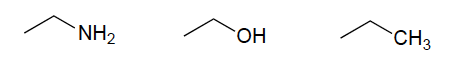Solution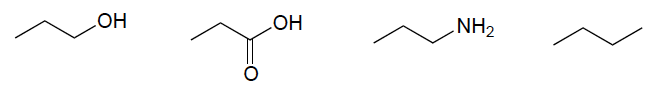Solution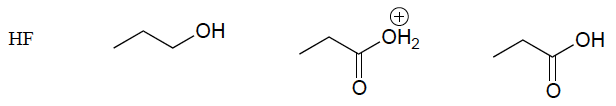Solution

Rank these molecules in terms of decreasing basicity: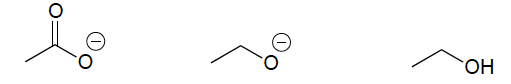Solution

What is the most acidic group of protons on methyl acetate? (This is a flammable liquid with a smell reminiscent of some glues and nail polish.)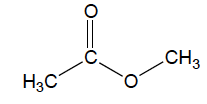Solution

From the structures below, what is the strongest acid? What is the strongest base?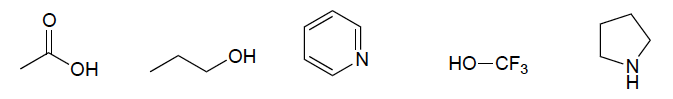Solution

Show the structures of species X and Y in the following acid-base reactions.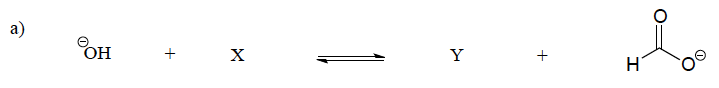Solution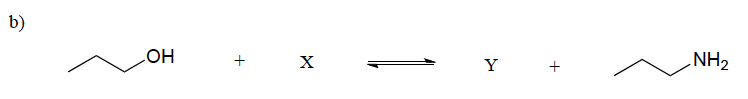Solution

Classify the solutions as acidic or basic.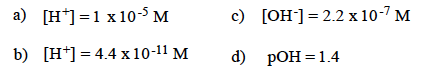Solution

Rank these acids from weakest to strongest:

CF3COOH HCO3- H2PO4- CH3COOH

Solution

What structural features of a molecule may effect the pKa of an acid?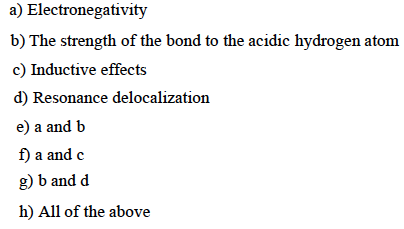Solution

Which of the following is the strongest acid?

CH3OH CH3SH CH3PH2

Solution

Which of the following is the strongest base?

CH3NH- CH3O- F-

Solution

Heartburn is caused by a buildup of excessive amounts of stomach acid, particularily HCl. This acid is used to digest the food we eat, but it can often back up into the esophagus causing that burning sensation many of us are familiar with. The symptoms of heartburn can be treated with a mild base, which acts to neutralize the excess HCl. For example, Tums is a very commonly used antacid that can be bought over the counter. Other antacids, such as Alka Seltzer, work in similar ways. Below, show the mechanism and products for reaction in which calcium carbonate (the active ingredient in Tums) neutralizes HCl in a simple proton transfer reaction. Following this acid-base reaction, carbonic acid quickly degrades into CO2 and H2O.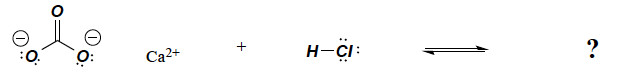Solution

Identify the more acidic compound in the following pairs: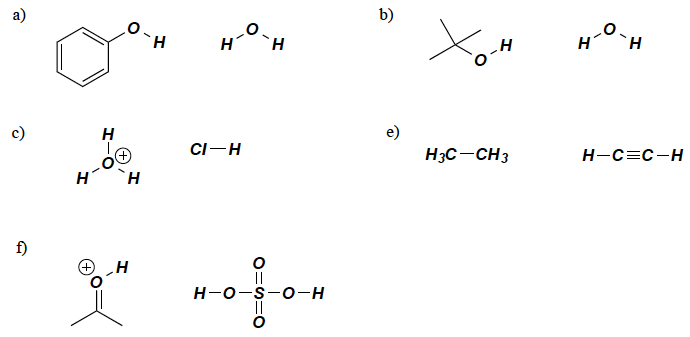Solution

Using the concept of resonance delocalization of charge, find which compound is the stronger base.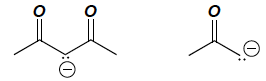Solution

Using a pKa table, identify the stronger base in each pair of compounds.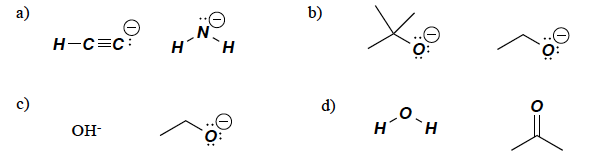Solution

Determine which proton is more acidic.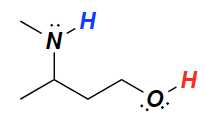Solution

Determine which proton is more acidic using resonance arguments.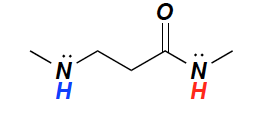Solution

Determine which proton is more acidic and explain how you arrived at your answer.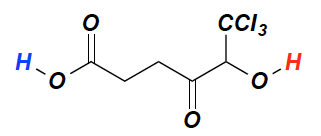Solution

List the following carboxylic acids in order of decreasing acidity.a. How does the presence of an electronegative substituent such as Cl affect the acidity of a carboxylic acid?

b. How does the location of the substituent affect the acidity of the carboxylic acid?

Solution

For each of the following compounds, draw the form in which it will predominate at pH =3, pH =6, pH =10, and pH =14.Solution

Which compound has the lower pKa?Solution

For each pair of compounds below, indicate which is more acidic.Solution

Write the formula for the conjugate base of each of the following acids:

a. sulfurous acid, H2SO3 b. chloric acid, HClO3 c. hydrogen sulfide, H2S

d. dimethyloxonium, (CH3)2OH+ e. hydrogen sulfate, HSO4-

Solution

Write the formula for the conjugate acid of each of the following bases:

a. dimethylamide, (CH3)2N- b. sulfide, S2- c. ammonia, NH3

d. acetone, (CH3)2NH e. 2,2,2-triflouroethoxidev, CF3CH2O-

Solution

Which is the stronger acid, nitrous (HNO2 pKa = 3.3) or phosphorous acid (H3PO3 pKa = 1.3)?

Calculate the Ka for each.

Solution

Show, using resonance, why acetic acid is more acidic than methanol.

Solution

For each of the following compounds, shown in their acidic forms, write the form that will predominate in a solution of pH = 5.5.Solution

A naturally occuring amino acid such as alanine has both a carboxylic acid group (pKa = 2.34) and an amine group (pKa = 9.69).a. Draw the structure of alanine in a solution at physiological pH (pH = 7.3).

b. Is there a pH at which neither functional group will have a formal charge?

c. At what pH will alanine have no net charge ( the amount of negative charge will be the same as the amount of positive charge)?

Solution

Indentify the strongest acid and the weakest acid in each row:Solution

Indicate the order of acid strength of these compounds by ranking 1-4 (1 being the strongest acid):

*note: C6H5 - phenyl groupSolution

To the following compounds:

a) Indicate the order of acid strength by ranking 1-4 (1 being the strongest acid):

b) Find the most acidic C-H proton of each:

*note: C6H5 - phenyl groupSolution

Indicate the order of base strength of these compounds by ranking 1-4 (1 being the strongest base):Determine the structure(s) of the most favored conjugate base.Solution